Processing ......FreeComputerBooks.com Links to Free Computer, Mathematics, Technical Books all over the World

Elementary Number Theory: Primes, Congruences, and Secrets: A Computational Approach
Top Free C++ Books 🌠 - 100% Free or Open Source!
• Title Elementary Number Theory: Primes, Congruences, and Secrets: A Computational Approach
• Author(s) William Stein
• Publisher: Springer 2009 edition (December 3, 2008); eBook (Updated on January 23, 2017)
• Hardcover/Paperback 168 pages
• eBook PDF (172 pages, 2.6 MB)
• Language(s): English and Korean
• ISBN-10: 0387855246
• ISBN-13: 978-0387855240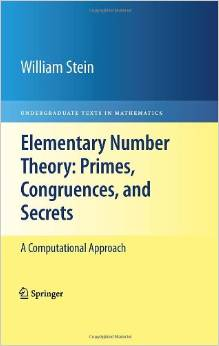Book Description

This book is an introduction to elementary number theory with a computational flavor. Many numerical examples are given throughout the book using the SageMath mathematical software. The text is aimed at an undergraduate student with a basic knowledge of groups, rings and fields. Each chapter concludes with several exercises.

It's all about prime numbers, congruences, secret messages, and elliptic curves that you can read cover to cover. It grew out of undergruate courses that the author taught at Harvard, UC San Diego, and the University of Washington.

The systematic study of number theory was initiated around 300B.C. when Euclid proved that there are infinitely many prime numbers. At the same time, he also cleverly deduced the fundamental theorem of arithmetic, which asserts that every positive integer factors uniquely as a product of primes.

Over 1000 years later (around 972A.D.) Arab mathematicians formulated the congruent number problem that asks for a way to decide whether or not a given positive integer n is the area of a right triangle, all three of whose sides are rational numbers.

Then another 1000 years later (in 1976), Diffie and Hellman introduced the first ever public-key cryptosystem, which enabled two people to communicate secretly over a public communications channel with no predetermined secret; this invention and the ones that followed it revolutionized the world of digital communication.

In the 1980s and 1990s, elliptic curves revolutionized number theory, providing striking new insights into the congruent number problem, primality testing, public-key cryptography, attacks on public-key systems, and playing a central role in Andrew Wiles' resolution of Fermat's Last Theorem.

• William Stein is an Associate Professor of Mathematics at the University of Washington. He is also the author of Modular Forms, A Computational Approach (AMS 2007), and the lead developer of the open source software, Sage.
Reviews, Ratings, and Recommendations: Related Book Categories: Read and Download Links:Similar Books:
•A Computational Introduction to Number Theory and Algebra

This introductory book emphasises algorithms and applications, such as cryptography and error correcting codes, and is accessible to a broad audience. The coverage includes the basics of number theory, abstract algebra and discrete probability theory.

•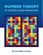Number Theory: In Context and Interactive (Karl-Dieter Crisman)

This is a textbook for an upper-level number theory course, with a clear vision to expose students to the connections to all areas of mathematics, and nearly every concept can be visualized or experimented with using the mathematics software SageMath.

•An Introduction to the Theory of Numbers (Leo Moser)

This book, which presupposes familiarity only with the most elementary concepts of arithmetic (divisibility properties, greatest common divisor, etc.), is an expanded version of a series of lectures for graduate students on elementary number theory.

•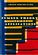Number Theory and Its Applications (Cheon Seoung Ryoo)

Number theory plays a fundamental and important role in mathematics and applied mathematics. This book is based on recent results in all areas related to number theory and its applications.

•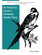An Introductory Course in Elementary Number Theory (Wissam Raji)

This book contains a useful introduction to important topics that need to be addressed in a course in number theory. Proofs of basic theorems are presented in an interesting and comprehensive way that can be read and understood even by non-majors.

•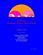Yet Another Introductory Number Theory Textbook (J. A. Poritz)

This introductory number theory textbook has a particular emphasis on connections to cryptology. It broaches the subject of these cryptologic algorithms to develop connections to the topic of number theory.

•Topology of Numbers (Allen Hatcher)

A textbook on elementary number theory from a geometric point of view, as opposed to the usual strictly algebraic approach. A fair amount of the book is devoted to studying Conway's topographs associated to quadratic forms in two variables.

•Magic Squares and Cubes (William Symes Andrews)

This book cover topics such as magic squares, magic cubes, the Franklin squares, magics and Pythagorean numbers, the theory of reversions, magic circles, spheres, and stars, and magic octahedroids, among other things.

•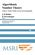Algorithmic Number Theory: Lattices, Curves, and Cryptography

This text provides a comprehensive introduction to algorithmic number theory for beginning graduate students, written by the leading experts in the field. It includes several articles that cover the essential topics in this area.

•A Course in Algebraic Number Theory (Robert B. Ash)

This book explores the general theory of factorization of ideals in Dedekind domains as well as the number field case. Detailed calculations illustrate the use of Kummer's theorem on lifting of prime ideals in extension fields.

•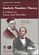Analytic Number Theory: A Tribute to Gauss and Dirichlet

The book begins with a definitive summary of the life and work of Dirichlet and continues with thirteen papers by leading experts on research topics of current interest in number theory that were directly influenced by Gauss and Dirichlet.

•Essays on the Theory of Numbers (Richard Dedekind)

This book contains the two most important essays on the logical foundations of the number system by the famous German mathematician J. W. R. Dedekind. The contents of these essays belong to the foundations of mathematics and our number system.

Book Categories
 :All CategoriesTop Free BooksRecent BooksMiscellaneous BooksComputer EngineeringComputer LanguagesComputer ScienceData Science/DatabasesJava and Java EE (J2EE)Linux and UnixMathematicsMicrosoft and .NETMobile ComputingNetworking and CommunicationsSoftware EngineeringSpecial TopicsWeb Programming
Other Categories